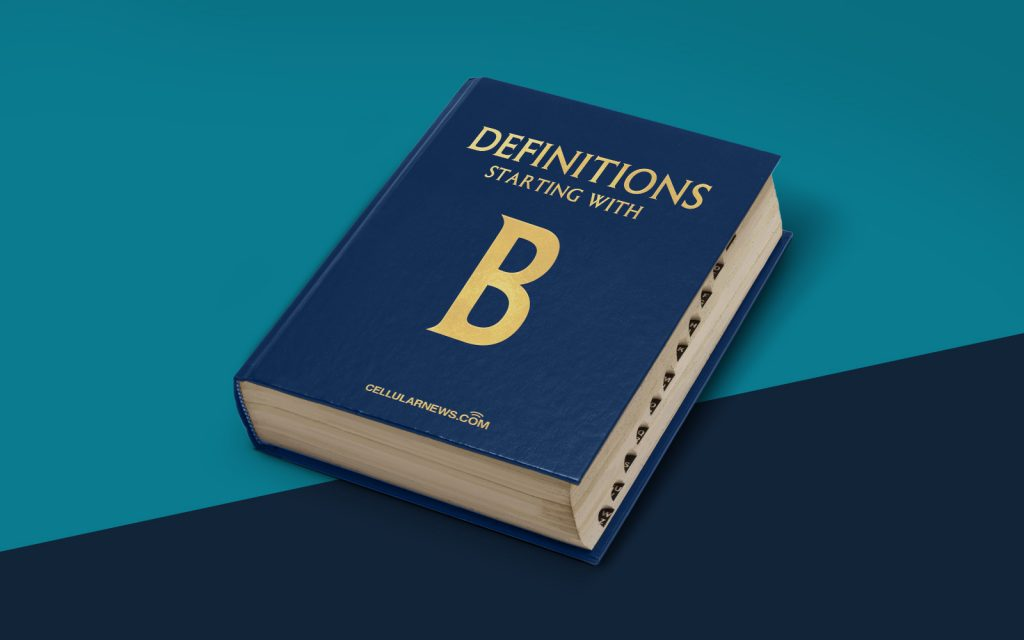Definitions## What is a Binary-Coded Decimal (BCD)? Defining the Basics

Welcome to our “Definitions” category, where we unravel the complex jargon of the digital world. Today, we dive into the world of Binary-Coded Decimal, or BCD for short. As an SEO expert, I strive to provide you with an informative and engaging blog post that not only answers your questions but also ranks high on search engines like Google. So, without further ado, let’s demystify the world of BCD!

## Key Takeaways:

• BCD is a specialized form of representing decimal numbers in a binary format.
• Each decimal digit is represented by a four-bit binary code, allowing for easy conversion between binary and decimal.

## Unraveling the Mystery of BCD

Binary-Coded Decimal, or BCD, is a system used to represent decimal numbers in a binary format. In simple terms, BCD allows for the easy conversion between binary and decimal values, making it a useful tool in digital systems. But what exactly makes BCD different from regular binary representation? Let’s break it down:

1. Decimal Representation: In our day-to-day lives, we are accustomed to using a decimal number system, where numbers are represented using the digits 0-9. For example, the number 25 in decimal representation is a combination of the digit 2 (tens place) and the digit 5 (ones place).
2. Binary Representation: On the other hand, computers primarily use a binary number system, where numeric values are represented using only 0s and 1s. The number 25 in binary representation would be written as 11001.

Now, here’s where Binary-Coded Decimal comes into play. Rather than using a direct binary representation, BCD maps each decimal digit to a unique four-bit binary code. This means that each decimal digit from 0 to 9 can be represented using four bits. For example:

• The decimal digit 0 is represented as 0000 in BCD.
• The decimal digit 1 is represented as 0001 in BCD.
• And so on, until the decimal digit 9, which is represented as 1001 in BCD.

This organized mapping of decimal digits in BCD allows for easy conversion between binary and decimal representations. It simplifies arithmetic calculations and digital circuit designs, making it a valuable tool in various applications such as calculators, digital displays, and data encoding.

## Key Takeaways:

• BCD is a specialized form of representing decimal numbers in a binary format.
• Each decimal digit is represented by a four-bit binary code, allowing for easy conversion between binary and decimal.

So, the next time you encounter the term Binary-Coded Decimal, you can confidently say that it is a system that represents decimal numbers using binary codes. With its ease of conversion and practical applications, BCD adds a touch of simplicity to the complex world of binary operations. We hope this article has shed some light on the definition and functionality of BCD – another definition demystified on our “Definitions” page!

Close
Latest Articles